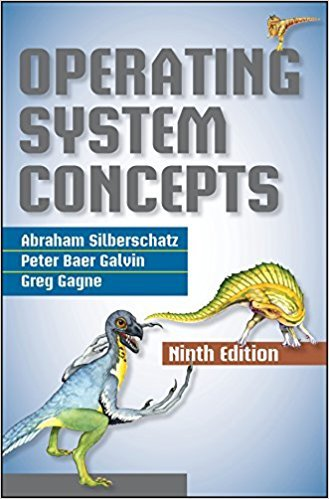×
×

# Consider two processes, P1 and P2, where p1 = 50, t1 = 25, p2 = 75, andt2 = 30.a. CanISBN: 9781118063330 327

## Solution for problem 6.31 Chapter 6

Operating System Concepts | 9th Edition

• Textbook Solutions
• 2901 Step-by-step solutions solved by professors and subject experts
• Get 24/7 help from StudySoup virtual teaching assistantsOperating System Concepts | 9th Edition

4 5 1 249 Reviews
11
1
Problem 6.31

Consider two processes, P1 and P2, where p1 = 50, t1 = 25, p2 = 75, andt2 = 30.a. Can these two processes be scheduled using rate-monotonicscheduling? Illustrate your answer using a Gantt chart such asthe ones in Figure 6.16Figure 6.19.b. Illustrate the scheduling of these two processes using earliestdeadline-first(EDF) scheduling.

Step-by-Step Solution:
Step 1 of 3

EXSC223:Weekof9/12/16 NotesfromthetextbookCh.3.10 3.10Thecellcycleconsistsofinterphaseandamitoticphase • Cellcycle:changesacellgoesthroughfromwhenitisformedtowhenitreproduces • Twomajorperiodsforthecell o Interphase:cellgrowsandcarriesoutnormalactivities...

Step 2 of 3

Step 3 of 3

##### ISBN: 9781118063330

Unlock Textbook Solution